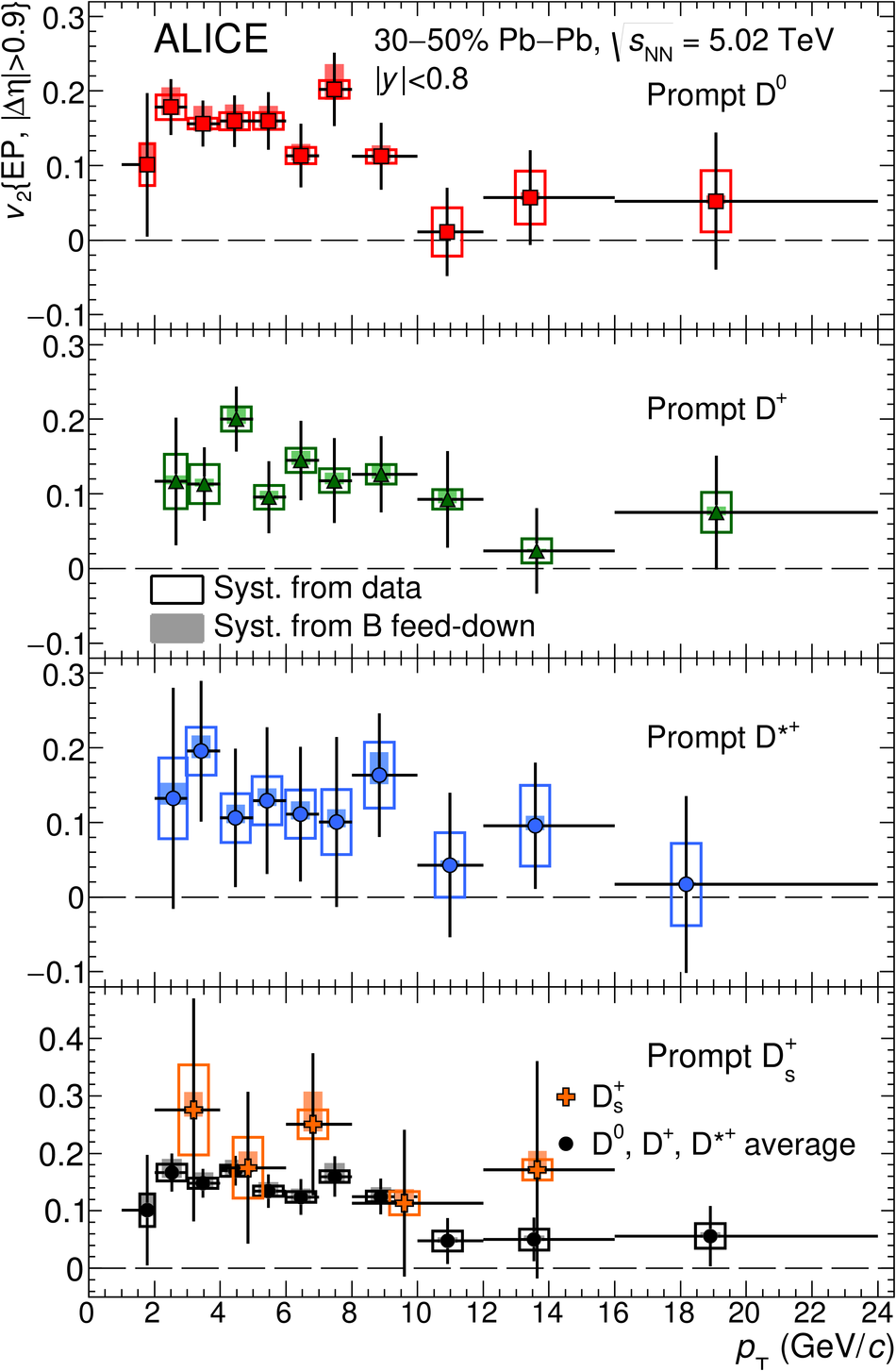# D-meson azimuthal anisotropy in mid-central Pb-Pb collisions at $\mathbf{\sqrt{s_{\rm NN}}=5.02}$ TeV

The azimuthal anisotropy coefficient $v_2$ of prompt D$^0$, D$^+$, D$^{*+}$ and D$_s^+$ mesons was measured in mid-central (30-50% centrality class) Pb-Pb collisions at a centre-of-mass energy per nucleon pair $\sqrt{s_{\rm NN}} = 5.02$ TeV, with the ALICE detector at the LHC. The D mesons were reconstructed via their hadronic decays at mid-rapidity, $|y|<~0.8$, in the transverse momentum interval $1<~p_{\rm T}<~24$ GeV/$c$. The measured D-meson $v_2$ has similar values as that of charged pions. The D$_s^+$ $v_2$, measured for the first time, is found to be compatible with that of non-strange D mesons. The measurements are compared with theoretical calculations of charm-quark transport in a hydrodynamically expanding medium and have the potential to constrain medium parameters.

Figures

## Figure 1

 Elliptic flow coefficient as a function of $\pt$ for prompt $\Dzero$, $\Dplus$,$\Dstar$ and $\Ds$ mesons and their charge conjugates for $\PbPb$ collisions in the centrality class 30--50\% The bottom panel also shows the average$v_2$ of $\Dzero$, $\Dplus$ and $\Dstar$. The symbols are positionedhorizontally at the average $\pt$ of the reconstructed D mesons Vertical error bars represent the statistical uncertainty, empty boxes the systematicuncertainty associated with the D-meson anisotropy measurement and the event-planeresolution. Shaded boxes show the feed-down uncertainty.## Figure 2

 Average of $\Dzero$, $\Dplus$ and $\Dstar$ $v_2$ as a function of$\pt$ at $\sqrtsNN=5.02~\tev$, compared with the same measurement at $\sqrtsNN=2.76~\tev$~ and to the $\pi^\pm$ $v_2$ measured with the EP method~ and with the scalar production (SP) method~.## Figure 3

 Average of $\Dzero$, $\Dplus$ and $\Dstar$ $v_2$ as a function of$\pt$, compared with modelcalculations~ For MC@sHQ+EPOS2 and TAMU the band represents theuncertainty of the calculation.